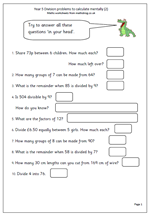# Year 5 division problems: calculating mentallyHere is the second page which looks at Year 5 division problems that can be answered by using mental skills. The questions cover a range of concepts involving division, including:

remainders

divisibility rule for 9

and factors.

Watch out for errors that occur in questions such as number 9, where the number of whole lengths of wire is calculated and the remainder is irrelevant.

This page, and other similar worksheets can be found in the Year 5 calculating category.

Division problems (2)